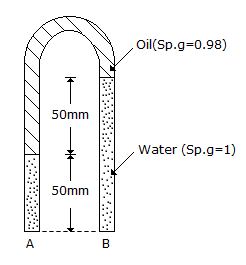# Civil Engineering - UPSC Civil Service Exam Questions

46.

In a three-layered soil, water flows parallel to stratification. The thickness of the middle layer is twice that of top and bottom layer. The coefficient of permeability of middle layer (2K) is twice that of top and bottom layer (K). What is the average coefficient of permeability for this flow ?

 A. K B. 1.33 K C. 1.5 K D. 0.66 K

Explanation:

No answer description available for this question. Let us discuss.

47.

Which type of brick masonry bond is provided for heavy loads on masonry ?

 A. English bond B. Zigzang bond C. Single Flemish bond D. Double Flemish bond

Explanation:

No answer description available for this question. Let us discuss.

48.

In the set up shown in the above figure, assuming the specific weight of water as 10, 000 N/m3, the pressure difference between the points A and B will beA. 10 N/m2 B. - 10 N/m2 C. 20 N/m2 D. -20 N/m2

Explanation:

No answer description available for this question. Let us discuss.

49.

The ratio of the "standard error of a single observation of unit weight" to the standard error of the arithmetic mean of 'n' observation all of unit weight, will be

 A. (1/n) B. n2 C. 3n D. n

Explanation:

No answer description available for this question. Let us discuss.

50.

If the azimuths of the two tangents to a circular curve of radius 100 m are due north and due east, then the area bounded by the two tangents and the circular curve will be

 A. 7857 sq. m B. 5000 sq. m C. 3143 sq. m D. 2143 sq. m